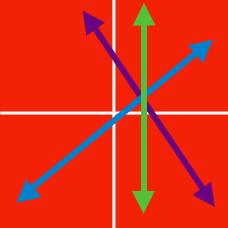Algebra

System of Linear Equations - Elimination

Solve the following system of equations: $\begin{cases} 2x+y=7 \\ 4x-y=5. \end{cases}$

Solve the following system of equations: $\begin{cases} -3x+4y=29 \\ -7x-4y=1. \end{cases}$

Let $x = a$ and $y = b$ be the solution of the following system of linear equations: $x-y=7,\quad 3x+2y=26.$ What is the value of $a+b$?

Given that $x$ and $y$ are real numbers satisfying the equations $3x - 2y = 22$ and $7x + 3y = 105$, what is the value of $x+y$?

Solve the following system of equations: $\begin{cases} 6x+2y=42 \\ 8x-4y=36. \end{cases}$

×